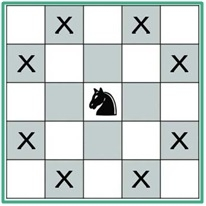# The Knight’s tour problem

Data StructureBacktracking AlgorithmsAlgorithms

In chess, we know that the knight can jump in a special manner. It can move either two squares horizontally and one square vertically or two squares vertically and one square horizontally in each direction, So the complete movement looks like English letter ‘L’.In this problem, there is an empty chess board, and a knight starting from any location in the board, our task is to check whether the knight can visit all of the squares in the board or not. When It can visit all of the squares, then place the number of jumps needed to reach that location from the starting point.

This problem can have multiple solutions, but we will try to find one possible solution.

## Input and Output

Input:
The size of a chess board. Generally, it is 8. as (8 x 8 is the size of a normal chess board.)
Output:
The knight’s moves. Each cell holds a number, that indicates where to start and the knight will reach a cell at which move.

0  59  38  33  30  17   8  63
37  34  31  60   9  62  29  16
58   1  36  39  32  27  18   7
35  48  41  26  61  10  15  28
42  57   2  49  40  23   6  19
47  50  45  54  25  20  11  14
56  43  52   3  22  13  24   5
51  46  55  44  53   4  21  12

## Algorithm

isValid(x, y, solution)

Input − Place x and y and the solution matrix.

Output − Check whether the (x,y) is in place and not assigned yet.

Begin
if 0 ≤ x ≤ Board Size and 0 ≤ y ≤ Board Size, and (x, y) is empty, then
return true
End

knightTour(x, y, move, sol, xMove, yMove)

Input − (x, y) place, number of moves, solution matrix, and possible x and y movement lists.

Output − The updated solution matrix if it exists.

Begin
if move = Board Size * Board Size, then //when all squares are visited
return true
for k := 0 to number of possible xMovement or yMovement, do
xNext := x + xMove[k]
yNext := y + yMove[k]
if isValid(xNext, yNext, sol) is true, then
sol[xNext, yMext] := move
if knightTour(xNext, yNext, move+1, sol, xMove, yMove), then
return true
else
remove move from the sol[xNext, yNext] to backtrack
done
return false
End

## Example

#include <iostream>
#include <iomanip>
#define N 8

using namespace std;
int sol[N][N];

bool isValid(int x, int y, int sol[N][N]) {     //check place is in range and not assigned yet
return ( x >= 0 && x < N && y >= 0 && y < N && sol[x][y] == -1);
}

void displaySolution() {
for (int x = 0; x < N; x++) {
for (int y = 0; y < N; y++)
cout << setw(3) << sol[x][y] << " ";
cout << endl;
}
}

int knightTour(int x, int y, int move, int sol[N][N], int xMove[N], int yMove[N]) {
int xNext, yNext;
if (move == N*N)     //when the total board is covered
return true;

for (int k = 0; k < 8; k++) {
xNext = x + xMove[k];
yNext = y + yMove[k];
if (isValid(xNext, yNext, sol)) {     //check room is preoccupied or not
sol[xNext][yNext] = move;
if (knightTour(xNext, yNext, move+1, sol, xMove, yMove) == true)
return true;
else
sol[xNext][yNext] = -1;// backtracking
}
}
return false;
}

bool findKnightTourSol() {
for (int x = 0; x < N; x++)     //initially set all values to -1 of solution matrix
for (int y = 0; y < N; y++)
sol[x][y] = -1;
//all possible moves for knight
int xMove = {  2, 1, -1, -2, -2, -1,  1,  2 };
int yMove = {  1, 2,  2,  1, -1, -2, -2, -1 };
sol  = 0;     //starting from room (0, 0)

if (knightTour(0, 0, 1, sol, xMove, yMove) == false) {
cout << "Solution does not exist";
return false;
} else
displaySolution();
return true;
}

int main() {
findKnightTourSol();
}

## Output

 0  59  38  33  30  17   8  63
37  34  31  60   9  62  29  16
58   1  36  39  32  27  18   7
35  48  41  26  61  10  15  28
42  57   2  49  40  23   6  19
47  50  45  54  25  20  11  14
56  43  52   3  22  13  24   5
51  46  55  44  53   4  21  12